# A Quasiclassical trajectory calculation to compute the reaction cross section and thermal rate constant for the cesium exchange reaction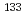CsI +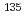Cs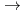Cs + ICs
One of important research targets in the development of cesium isotope separation system is design of recovery process of cesium atom. Relevant to this research target, the reaction cross section and reaction rate constant of a cesium exchange reaction through collision of the cesium iodide molecules with cesium atoms are calculated by a quasi-classical trajectory calculation based on a potential energy surface obtained by quantum chemistry calculations. Consequently, the rate constant is calculated to be 3.6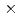10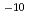cm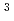molecule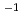s, as large as collision rate in the present condition. In addition, slightly positive temperature dependence is observed in the rate constant. This behavior is explained with the long-range attractive force and effect of subsequent dissociation process.